﻿ 基于排队论的机场出租车调度问题研究

# 基于排队论的机场出租车调度问题研究Taxi Assignment Problem in the Airport Based on Queue Theory

Abstract: In this paper, the taxi assignment problem in the airport is studied based on queue theory. Firstly, we consider all the corresponding factors of decision-making of taxi-driver and the mechanics of this problem in a whole, and give one mathematical model for the drivers based on queue theory, as well as the strategies under different conditions. Secondly, we give some optimization for the taxi assignment system in the airport based on the model mentioned above, and establish one model named multi-point single-assignment system. Compare with the data from Pudong Airport, our model is relatively feasible and useful.

1. 引言

(A) 前往机场的“蓄车池”。由于需要排队等待排在前面的出租车离开和等待乘客前来乘车，因此，需要付出一定的时间成本来载客。

(B) 不前往“蓄车池”，直接空车返回市区拉客。但是会带来空载和损失掉可能存在的载客收益。

1) 构建一个关于出租车司机的决策模型，给出司机选择的策略；

2) 结合上海浦东机场的相关数据以及上海的出租车数量，根据获取的数据，确定司机的选择策略；

3) 对两条并行车道合理设置“上车点”，并对乘客和出租车进出“乘车区”进行合理安排，在保证司机和乘客的安全的条件下，实现整体的乘车效率达到最大；

4) 具体化“优先权”的规则，弥补载短途的司机的收益损失，让机场的出租车司机的收益尽量保持均衡。

2. 模型假设

1) 假设蓄车池规模无限大，允许车辆无限排队。

2) 假设不计出租车车重变化及突发路况急刹车等特殊情况对每公里油耗的变化。

3) 假设不考虑突发事件对乘客上车时间的影响。

4) 假设短途返程的司机均将乘客送达目的地，不存在中途放客。

3. 符号说明

4. 模型的建立与求解

4.1. 基于排队论的出租车司机决策模型

4.1.1. 影响出租车司机决策因素的机理分析

4.1.2. 决策因子的分析

1) 收益R

$R={S}_{f}×{R}_{s}=v×{t}_{1}×{R}_{s}.$ (1)

2) 空载费用E

$E={S}_{e}×u=v×{t}_{2}×u.$ (2)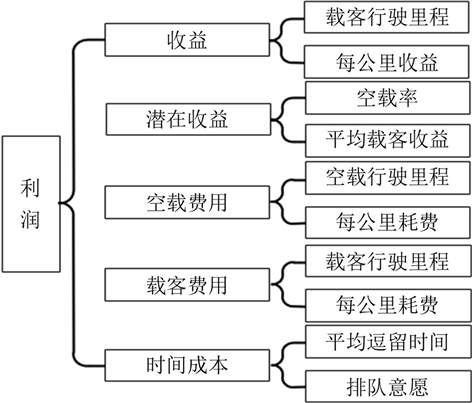Figure 1. Decision system diagram

3) 载客费用F

$F={S}_{f}×u=v×{t}_{1}×u.$ (3)

4) 潜在收益 ${R}_{q}$

${R}_{q}={E}_{l}×\stackrel{¯}{R}.$ (4)

5) 时间成本 ${C}_{t}$

${C}_{t}=\stackrel{¯}{T}×{R}_{t}.$ (5)

1) 输入过程

2) 排队规则

3) 服务台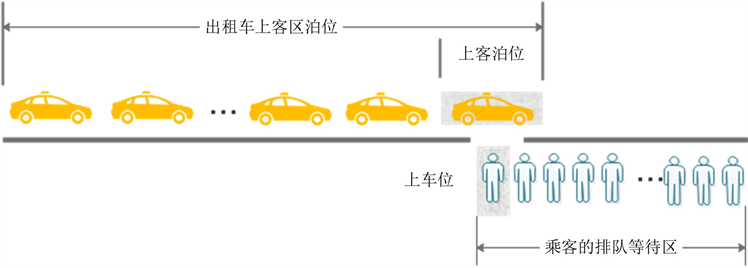Figure 2. Service desk diagram

${p}_{n}=P\left\{L=n\right\}\left(n=0,1,2,\cdots \right)$ 为出租车载客系统达到平衡状态后队长L的概率分布，由于每个状态下出租车到达上车点时间都是独立的，乘客上车时间同样也是独立的，因此有 ${\lambda }_{n}=\lambda ,n=0,1,2,\cdots$${\mu }_{n}=\mu ,n=0,1,2,\cdots$

$\rho =\frac{\lambda }{\mu }$ 由于在平稳状态下队长的分布为

${p}_{n}={C}_{n}{p}_{0},n=1,2,\cdots$ (6)

${C}_{n}=\frac{{\lambda }_{n-1}{\lambda }_{n-2}\cdots {\lambda }_{0}}{{\mu }_{n}{\mu }_{n-1}\cdots {\mu }_{1}},\text{\hspace{0.17em}}n=1,2,\cdots ,\text{\hspace{0.17em}}{p}_{0}=1-\rho .$ (7)

${p}_{n}={\rho }_{n}\left(1-\rho \right),\text{\hspace{0.17em}}\text{\hspace{0.17em}}n=1,2,\cdots$ (8)

${L}_{s}={\sum }_{n=0}^{\infty }n{p}_{n}={\sum }_{n=0}^{\infty }n{p}^{n}\left(1-\rho \right)=\frac{\rho }{1-\rho }=\frac{\lambda }{\mu -\lambda }.$ (9)

$P\left(\stackrel{¯}{T}>t\right)={\text{e}}^{-\left(\mu -\lambda \right)t},t\ge 0.$ (10)

$Q\left(n,N\right)={\text{e}}^{-\frac{n}{N}}.$ (11)

$\stackrel{¯}{T}=Q×\frac{1}{\mu -\lambda }.$ (12)

4.1.3. 司机选择决策模型的建立及策略的提出

$P=R-{C}_{t}-F-E-{R}_{q}.$ (13)

${P}_{A}={R}_{A}-{C}_{t}-{F}_{A}.$ (14)

${P}_{B}={R}_{B}-E-{F}_{B}-{R}_{q}.$ (15)

$Y=\left\{\begin{array}{l}\text{Plan}\text{\hspace{0.17em}}A,{P}_{A}\ge {P}_{B},\\ \text{Plan}\text{\hspace{0.17em}}B,{P}_{A}<{P}_{B}.\end{array}$ (16)

${P}_{A}={R}_{A}-\stackrel{¯}{T}{R}_{t}-{F}_{A}={S}_{fa}{R}_{s}-{\stackrel{¯}{R}}_{t}-u{S}_{fa}.$ (17)

${P}_{B}={R}_{B}-E-{F}_{B}{R}_{q}={S}_{fb}{R}_{s}-u{S}_{e}-u{S}_{fb}-{E}_{l}\stackrel{¯}{R}.$ (18)

${P}_{A}-{P}_{B}=\left({S}_{fa}-{S}_{fb}\right){R}_{s}+\left(-{S}_{fa}+{S}_{e}+{S}_{fb}\right)u-\stackrel{¯}{T}{R}_{t}+{\stackrel{¯}{E}}_{l}\stackrel{¯}{R}=v\left({t}_{1a}-{t}_{1b}\right){R}_{s}+\left(\stackrel{¯}{T}+{t}_{1b}-{t}_{1a}\right)uv-\stackrel{¯}{T}{R}_{t}+{E}_{l}\stackrel{¯}{R}.$ (19)

$\stackrel{¯}{T}+{t}_{1a}={t}_{2b}+{t}_{1b}.$ (20)

${P}_{A}-{P}_{B}=\stackrel{¯}{T}\left(uv-v{R}_{s}-{R}_{t}\right)+S{R}_{s}+{E}_{l}R.$ (21)

$\stackrel{¯}{T}>\frac{S{R}_{s}+{E}_{l}\stackrel{¯}{R}}{v{R}_{s}+{R}_{t}-uv}.$

4.2. 司机决策模型的实际应用与分析

4.2.1. 数据收集与处理Table 2. The data of relevant parameterTable 3. Number of passagers in Shanghai Pudong International Airport per month from 2015 to 2018

4.2.2. 上海浦东机场出租车司机选择方案的确立

${P}_{A}-{P}_{B}=T×271.19+242.446.$ (22)

${P}_{A}-{P}_{B}=\frac{{\text{e}}^{-\frac{n}{N}}}{187.5-186.9}×271.19+242.446.$ (23)Table 4. Circle value of storage tank of cars every month

4.2.3. 模型对相关因素的依赖性和模型合理性评价

1) 在不改变其他因素数据的情况下，选择一个二级指标进行改动。

2) 由于司机在做出决策时考虑的是将利润最大化，所以我们分别求出指标改动后的方案A利润PA和方案B利润PB进行作差，得出方案A较方案B的利润差。

3) 计算方案A较方案B利润的相对变动，并将其与二级指标数据的相对变动做比，得到利润对该指标变动的敏感系数，推导模型对该指标的依赖性。

Table 5. Initial value of index

$L=\frac{\left({P}_{A}^{1}-{P}_{B}^{1}\right)-{P}_{A}-{P}_{B}}{{P}_{A}-{P}_{B}}.$ (24)

$\eta =\frac{L}{20%}.$ (25)Table 6. Sensitivity coefficient of profit of plan A to various factors compared with plan B

4.3. 上车点及发车模式的确立

4.3.1. 两点依次发车模式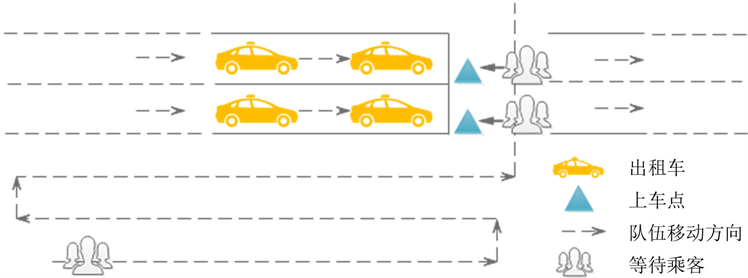Figure 3. Two points taxi queuing system

4.3.2. 多点单独发车模式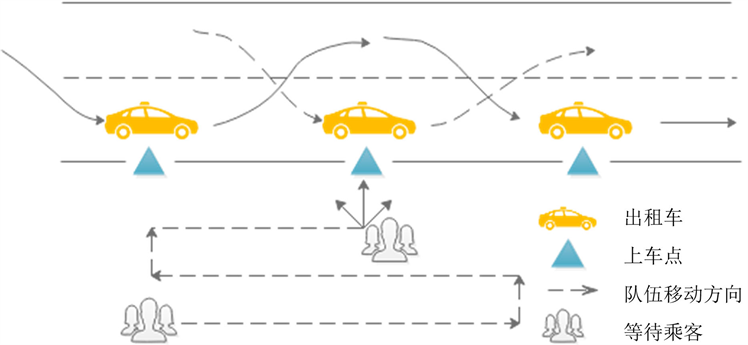Figure 4. Multi points taxi queuing system

$\rho =\frac{\lambda }{n\mu }<1.$ (26)

$\left\{\begin{array}{l}{P}_{0}={\sum }_{k=0}^{n-1}\frac{1}{k!}{\left[{\left(\frac{\lambda }{\mu }\right)}^{k}+\frac{1}{n!}\frac{1}{1-\rho }{\left(\frac{\lambda }{\mu }\right)}^{n}\right]}^{-1},\\ {P}_{m}=\left\{\begin{array}{l}\frac{{\lambda }^{m}}{{\mu }^{m}m!}{P}_{0},1\le m\le n,\\ \frac{1}{n!{n}^{m-n}}{\left(\frac{\lambda }{\mu }\right)}^{m},m>n.\end{array}\end{array}$ (27)

${L}_{s}=\frac{{\left(np\right)}^{n}\rho }{n!{\left(1-\rho \right)}^{2}}{P}_{0}.$ (28)

$\begin{array}{l}\mathrm{min}C\left(n\right)={C}_{1}+{C}_{2}=a{L}_{s}\left(n\right)+bn\\ \text{s}\text{.t}\text{.}\text{\hspace{0.17em}}\left\{\begin{array}{l}C\left(n\right)\le C\left(n+1\right),\\ C\left(n-1\right)\le C\left(n\right).\end{array}\end{array}$ (29)

${L}_{s}\left(n-1\right)-{L}_{s}\left(n\right)\ge \frac{a}{b}\ge {L}_{s}\left(n\right)-{L}_{s}\left(n+1\right).$ (30)Table 8. Data of cost model calculation process

4.4. 出租车短途返程“优先权”方案设计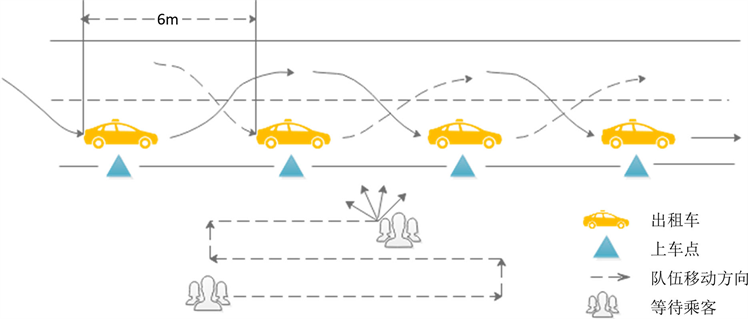Figure 5. Multi points taxi queuing system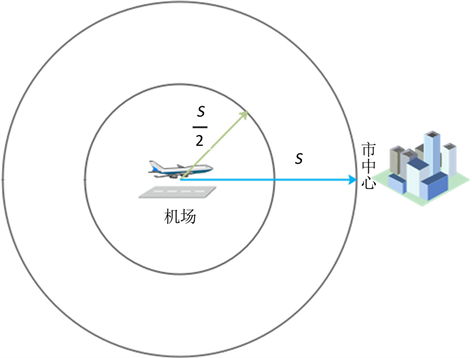Figure 6. Taxi driving range

${t}_{x}=\frac{S}{v}.$ (31)

5. 模型的评价

5.1. 优点

1) 本文所建立的模型均参考实际机场数据进行应用，可直观通过结果检验模型是否合理。

2) 将总的乘车效率最高问题转化为乘客平均等待时长和司机等待时长之和最小的指标规划问题，把握了主要矛盾的主要因素，舍弃了次要因素，从而简化了模型。

3) 在问题三中引入了排队论中的费用决策模型，对机场出租车接客系统进行优化，使得该系统中总的服务效率得到提升。

5.1. 缺点

1) 本文建立模型时考虑的情况皆适用于大规模机场，缺乏对机场规模较小情况的思考。

2) 在建立机场司机选择决策模型时，对于司机排队时间成本的计算固然合理，但仍有需要改进的地方。

6. 模型的评价

NOTES

*通讯作者。

 吴翊, 吴孟达, 成礼智. 数学建模的理论与实践[M]. 湖南: 国防科技大学出版社, 1999: 231-233.

 中国民用航空华东地区管理局. 华东地区机场运输生产情况[EB/OL]. http://hd.caac.gov.cn/HD_XXGK/HD_TJSJ/, 2016-04-27-2019-02-25.

 林思睿. 机场出租车运力需求预测技术研究[D]: [硕士学位论文]. 成都: 电子科技大学, 2018.

 广发证券. 公交II行业: 出租车深度报告[EB/OL]. https://doc.mbalib.com/view/4defa2e2a6e158cc1e649365a4ef40c0.html, 2015-09-18.

 姚飞. 公司利润敏感性分析及对策研究[D]: [硕士学位论文]. 哈尔滨: 黑龙江大学, 2018.

 姚丽君, 邵家玉. 基于地铁成本控制分析以提高运营效益的研究[J]. 科技资讯, 2010(32): 63-64.

 魏中华, 王琳, 邱实. 基于排队论的枢纽内出租车上客区服务台优化[J]. 公路交通科技(应用技术版), 2017, 13(10): 298-300.

 吴娇蓉, 李铭, 梁丽娟. 综合客运枢纽出租车上客点管理模式和效率分析[J]. 交通信息与安全, 2012, 30(4): 18-23.

 北京建筑工院. 汽车库建筑设计规范[M]. 北京: 中国建筑工业出版社, 2015.

 张隽, 关喜艳. 天河机场出租车短途返程优先配客[EB/OL]. http://hb.people.com.cn/n2/2019/0122/c194063-32559595.html, 2019-01-22.

Top